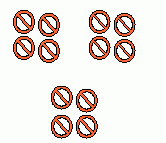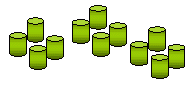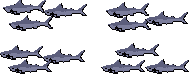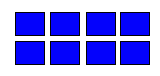## When It Comes To Math

Some fear it to death,

Some fall out of breath;

Here is a simple confess:

Math is not something worse

than giving birth…

Math Is cool

If you know the rule;

Math is fun

If you are its fan.

Keep trying,

You can do it without dying…

1+1=2,

Math says something perfectly true.

2+2=4,

You can make a satisfying score.

3+3=6,

Math has some magical tricks.

If you truly understand,

You will love math and feel grand.

*****

When i started blogging one year ago, I truly enjoyed doing Monday Math posts. Glad to give it a try again today.

Today’s Math problem:If it takes 12 people 3 days to build 72 meters of fence, how many meters of fence can 15 people build in one day?

Hint: compute how many meters of fence each person build each day applying the first 3 numbers, then it is easy to calculate using giving condition!

Pick one:

A: 2

B: 12

C: 20

D: 24

E:30

Good Luck! I Wonder who can do it?

## D Is 4 Division (Math)

D is 4 D-I-V-I-S-I-O-N,

Which makes math a lovely function.

Division is fun,

If u r its fan.

Any number is divided by 1,

The answer is the same one.

An even number is divided by 2,

U get half of the number, true!

An odd number is divided by 2,

U end up with a reminder, not cool,

Any number is divided by itself,

The answer is 1, what fun!

Division is the inverse of  multiplication,

Practice makes perfection.

If C is divided by A equal to B,

Then A times B is equal to C,

And B times A is also equal to C.

For instance,

100 is divided by 4 is 25,

4 times 25 is 100,

25 times 4 is also 100.

Division is tough,

If u don’t work hard enough…

I love divisions,

Because it is cool,

Work on the below versions,

Divisions love U!

*****

The poem is originally written by Jingle as an entry 4

ABC Wednesday, D Is 4 Division!!

The following practices come from Reference: http://www.kwiznet.com/

Practices 2 learn Divisions Method:

2. Count the pictures in each group.
3. Write the number of items in one group or the number of groups

Example:
In the picture 12 items are divided into 3 groups with ___ in each.This is made of 3 groups of 4 in each.

 Q 1: In the picture below 6 stars are divided into 3 groups with ___ in each group.3 2 1 Q 2: In the picture below 8 medals are divided into 4 groups with ___ in each group.2 4 3 Q 3: In the picture below 12 cylinders are divided into ___ groups with 4 cylinders in each group.6 4 3 Q 4: In the picture below 12 fishes are divided into 4 groups with ___ in each group.4 3 6 Q 5: In the picture below 8 rectangles are divided into 4 groups with ___ in each group.2 3 4 Q 6: In the picture below _____ hearts are divided into 4 groups with 5 in each group.21 24 22 20 Q 7: In the picture below 10 parallelograms are divided into 2 groups with ___ in each group.6 5 10 Q 8: In the picture below 9 boxes are divided into 3 groups with ___ in each group.3 6 4

## Beach Is A Great Place 2 ExploreMy name is Pitten.

My age is seven.

I love collecting seashells on the shore,

I enjoy playing the sand and more.

Beach is a great place to explore,

Beach time has improved my arithmetic score.

I know how to add, subtract, and even multiply,

It is as easy as an apply pie.

1 times 7 is 7,

Add 4 years I turn eleven.

2 times 7 is 14,

That’s when I become a real teen.

3 times 7 is 21,

I love watching the rising sun.

4 times 7 is 28,

Doing math unit is simply great.

5 times 7 is 35,

I enjoy leaning how to dive.

6 times 7 is 42,

I choose an ocean over a pool.

7 times 7 is 49,

Running barefoot is so much fun.

8 times 7 is 56,

Life is full of numerical tricks.

9 times 7 is 63,

Swimming near a beach makes me feel FREE.

My name is Pitten,

My age is 7,

I love collecting seashells on the shore,

I enjoy playing the sand and more.

Beach is a great place to explore,

Beach time has improved my arithmetic score…

*****

This is an entry for

ABC WednesdayB is 4 BEACH!

This is also an entry 4 Poetry Pantry

and 4 Monday’s Child

Math is NOT that hard to learn if one makes it fun and relaxing…I hope that you enjoy reading this!

Happy Thursday!

U Rock!

😉  😉   😉

## Solve A Math Problem!

Hello, how are you?

If you are interested in math, this problem is for you to work on and solve.

Then multiple by 102,

Then divide by 5,

Then subtract by 14,

What do you get?

_____
Please use two (2) words only to describe it.

Thank you in advance, have FUN. 🙂

PS:correct answer in number is 888, I required you to say it using two words, all entries are well done.

Other possible way to say it:

triple 8’s,

triple eight,

triple A’s

sounds great 🙂 🙂 :).

Thank you all for the number FUN.